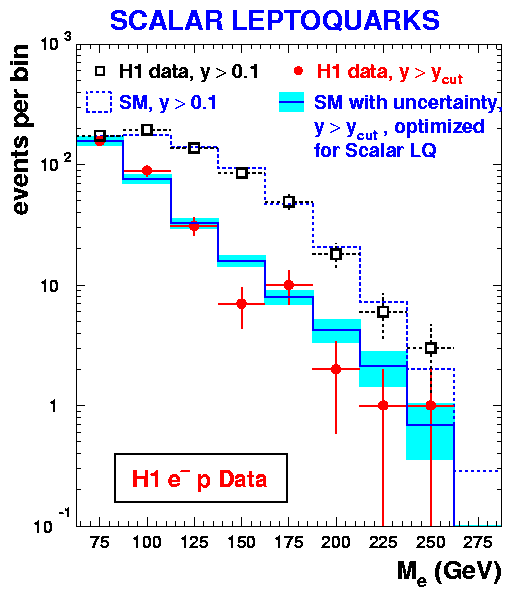# A Search for Leptoquark Bosons in Electron-Proton Collisions at HERA

Why are atoms electrically neutral ? While all chemical bindings and thus all life is based on this simple observation, the answer to this question is actually not so easy: Atoms are build of electrons of electrical charge -1, which move around a nucleus build of neutrons and protons which in turn consist of 3 quarks each. If these quarks carry a charge of exactly 1/3 or multiples of this number, then atoms can indeed be electrically neutral. But which law of nature should guarantee this exact relationship between the charges of elementary particles of such a different behaviour as electrons and quarks ? The answer might lie in theories beyond the well known Standard Model of particle physics, in so called Grand Unifying theories, which not only unify all forces of nature into one single theory, but also treat quarks and electrons as two members of the same family of particles and predict fixed relationships between them. While this appealing theory is by no means proven yet, there exists the possibility to detect further predictions of these theories, namely the possibility that electrons and quarks might fuse to a single particle and then separate again. The intermediate particle is named 'leptoquark'.The idea of this analysis is to directly produce these leptoquarks at the HERA collider by colliding electrons with quarks obtained from a beam of protons. The energies (E) of the electron and the quark then transform into the mass (M) according to Einsteins equation E=M c2, where c is the speed of light. Measuring the mass of the combined electron - quark system in a sample of such collisions yields a mass distribution as shown here. A leptoquark should show up as a significant peak on top of the distribution expected from Standard Model processes (the lines in the figure). Unfortunately no such peak is seen in the H1 data, so no leptoquarks are found.
Does this mean that the striking ideas about Grand Unification theories are wrong ? No, but if they exist they certainly cannot include leptoquarks with masses below 290 GeV, i.e. about 600,000 times the electron mass.

Last Update on 20/09/2001 by Peter Schleper (schleper@mail.desy.de)